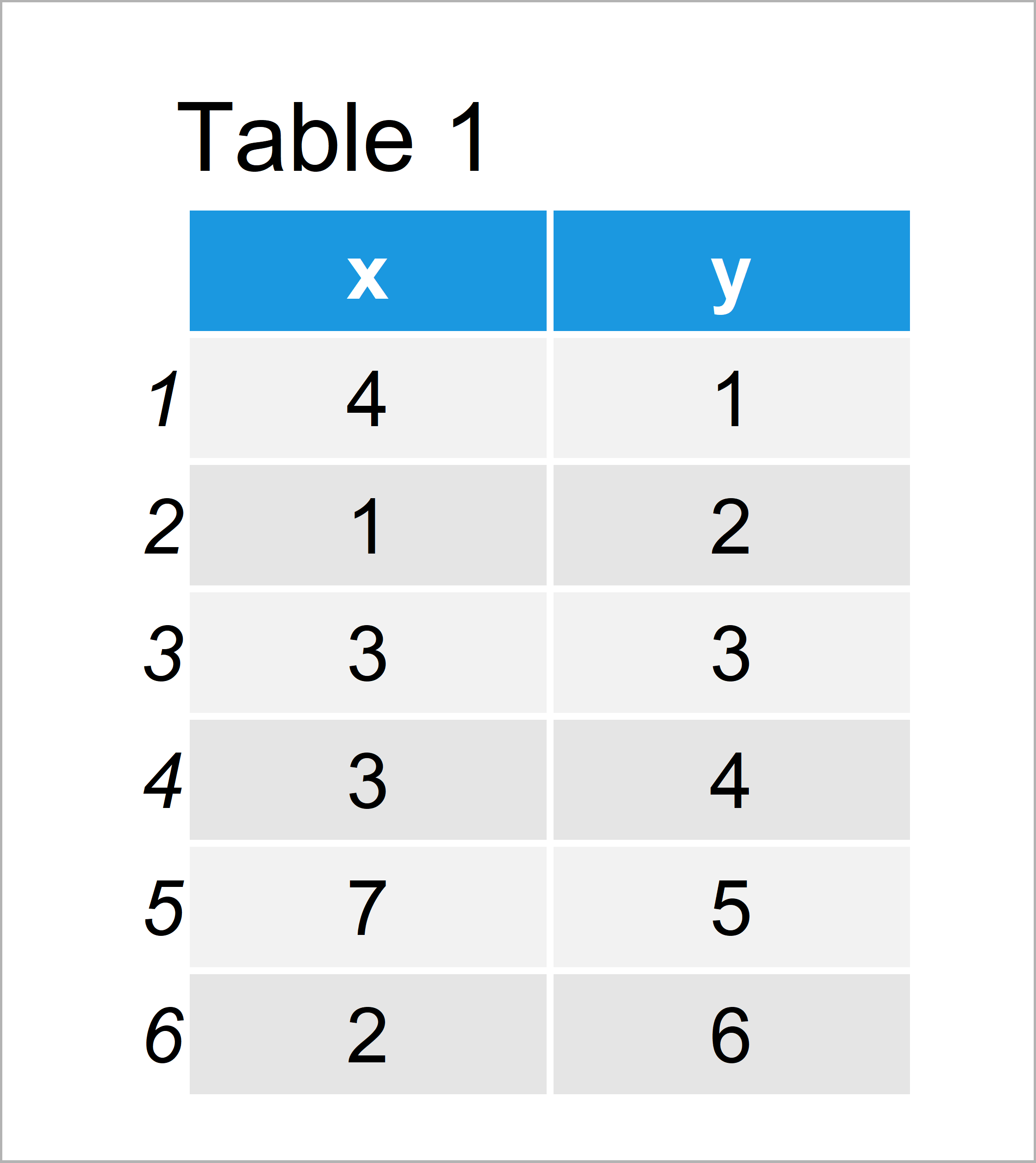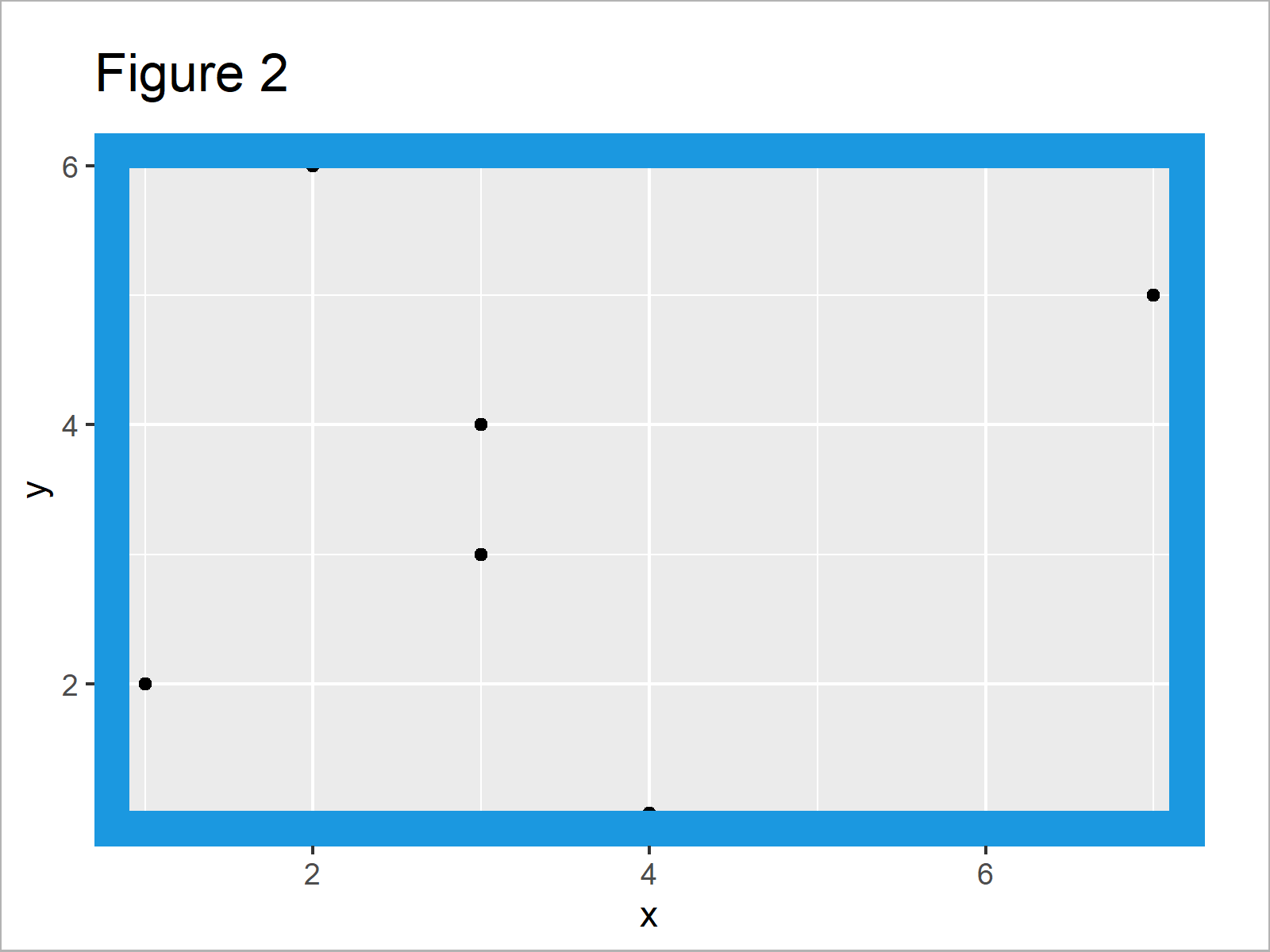# Add Panel Border to ggplot2 Plot in R (Example)

In this article you’ll learn how to draw a panel border to a ggplot graphic in the R programming language.

Let’s dive into it!

## Example Data, Add-On Packages & Default Plot

The first step is to create some data that we can use in the examples below:

```data <- data.frame(x = c(4, 1, 3, 3, 7, 2),    # Create example data
y = 1:6)
data                                           # Print example data```Table 1 shows the structure of our exemplifying data: It contains six rows and two columns.

We also have to install and load the ggplot2 package, to be able to use the corresponding functions:

```install.packages("ggplot2")                    # Install & load ggplot2
library("ggplot2")```

Now, we can plot our data as follows:

```ggp <- ggplot(data, aes(x, y)) +               # Create ggplot2 plot without panel border
geom_point()
ggp                                            # Draw ggplot2 plot without panel boarder```As shown in Figure 1, the previous syntax has plotted a ggplot2 scatterplot without any panel borders.

## Example: Draw Panel Border to ggplot2 Plot Using theme() Function & panel.border Argument

This example illustrates how to show a panel box around our ggplot2 plot. For this, we can use the theme function and the panel.border argument as shown below:

```ggp +                                          # Add panel border to ggplot2 plot
theme(panel.border = element_rect(color = "#1b98e0",
fill = NA,
size = 10))```In Figure 2 it is shown that we have created a ggplot2 plot with relatively thick blue panel borders using the previous R code.

Note that you can change the color and line width of our panel borders by modifying the color and size arguments within the element_rect function.

## Video, Further Resources & Summary

In case you need more information on the R programming codes of this article, I recommend watching the following video which I have published on my YouTube channel. In the video, I show the R code of this tutorial in R:

Furthermore, you might want to have a look at some of the other articles on my website.

In this R tutorial you have learned how to add panel borders to plots. If you have additional questions, don’t hesitate to let me know in the comments below.

Subscribe to the Statistics Globe Newsletter